DraftKings 2019 Big 10 Odds
+300
3 to 1Maryland
19.5% implied probability

+350
3.5 to 1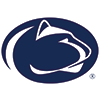Penn State
17.3% implied probability

+400
4 to 1Michigan State
15.6% implied probability

+700
7 to 1Iowa
9.7% implied probability

+700
7 to 1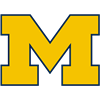Michigan
9.7% implied probability

+800
8 to 1Ohio State
8.6% implied probability

+1200
12 to 1Illinois
6.0% implied probability

+1200
12 to 1Wisconsin
6.0% implied probability

+2500
25 to 1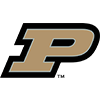Purdue
3.0% implied probability

+3500
35 to 1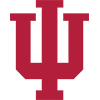Indiana
2.2% implied probability

+3500
35 to 1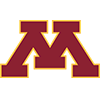Minnesota
2.2% implied probability

+50000
500 to 1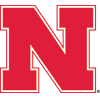Nebraska
0.2% implied probability

+50000
500 to 1Northwestern
0.2% implied probability